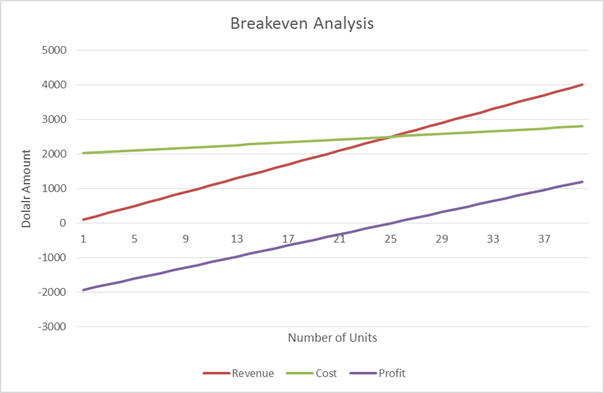Categories

# Breakeven Analysis

Breakeven Analysis is used to calculate the number of units you need to sell in order to cover all of your fixed and variable costs.

Equation

Break Even Analysis:

Fixed Costs / (Price per Unit – Cost per Unit) = Quantity to Produce

Quantity to Produce (Price per Unit – Cost per Unit) – Fixed Cost = Amount Earned

Example

• Item A is sold for \$100 per unit.
• Item A costs \$20 per unit
• Company X has fixed costs of \$2,000

The breakeven point is:

\$2,000 / (\$100 - \$20) = 25 units